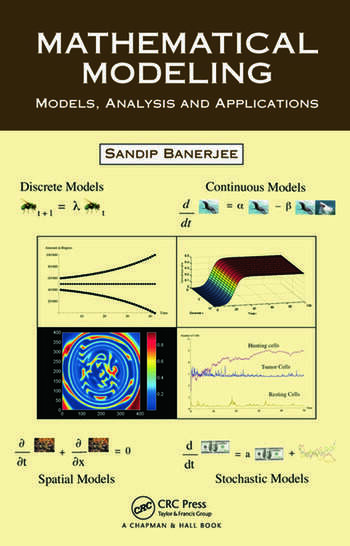# Mathematical Modeling: Models, Analysis and Applications

## 1st Edition

Sandip Banerjee

Chapman and Hall/CRC
Published February 7, 2014
Textbook - 276 Pages - 77 B/W Illustrations
ISBN 9781439854518 - CAT# K12528

For Instructors Request Inspection Copy

was \$110.00

USD\$88.00

SAVE ~\$22.00

FREE Standard Shipping!

## Preview

### Summary

Almost every year, a new book on mathematical modeling is published, so, why another? The answer springs directly from the fact that it is very rare to find a book that covers modeling with all types of differential equations in one volume. Until now. Mathematical Modeling: Models, Analysis and Applications covers modeling with all kinds of differential equations, namely ordinary, partial, delay, and stochastic. The book also contains a chapter on discrete modeling, consisting of differential equations, making it a complete textbook on this important skill needed for the study of science, engineering, and social sciences.

More than just a textbook, this how-to guide presents tools for mathematical modeling and analysis. It offers a wide-ranging overview of mathematical ideas and techniques that provide a number of effective approaches to problem solving. Topics covered include spatial, delayed, and stochastic modeling. The text provides real-life examples of discrete and continuous mathematical modeling scenarios. MATLAB® and Mathematica® are incorporated throughout the text. The examples and exercises in each chapter can be used as problems in a project.

Since mathematical modeling involves a diverse range of skills and tools, the author focuses on techniques that will be of particular interest to engineers, scientists, and others who use models of discrete and continuous systems. He gives students a foundation for understanding and using the mathematics that is the basis of computers, and therefore a foundation for success in engineering and science streams.

#### Instructors

We provide complimentary e-inspection copies of primary textbooks to instructors considering our books for course adoption.